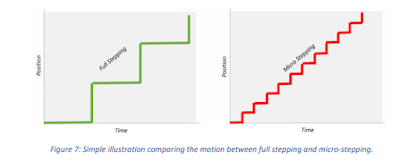## Saturday, October 27, 2018

### How to Choose A Suitable Rotation Speed Stepper Motor

While we chose a stepper motor online supplier , according to the speed, it is very to choose a motor working at the best situation. The stepper motor speed divided into :working at low speed, working at a more than 2000rpm speed and working at a wide range from low speed to high speed. The choice of stepper motor will be different.

While operated at a high speed, because of the influence of circuit inductance, the flow of the circuit current will be limited. Assuming that the coil reactance of the 1 phase is X, the frequency angular velocity of the driver current is w(electric angle), Its synchronous rotating rotor mechanical angle is wm, at high speed, the coil resistance R<<X, the impedance Z of the 1-phase winding becomes the following:

Z≈wL=NRwmL  (5)

When the rotor rotates at the rotation speed wm, the impedance Z requires that the number of teeth N of the rotor is as small as possible so that the current easily flows. In addition, the stator is driven by a constant current. If the rotational speed is too high, the cross-flow control cannot be achieved, and it will become a constant-voltage drive. When the rotation speed is high, this state is often driven. In this state, when the applied voltage is V, if The electromotive force is E, then its current I is determined by the following formula:

İ=(V(.)-E(.))/Z      (6)

Z=NrwmL

Figure 5.1 Equivalent circuit when rotating at high speed

The current I of the motor with small Nr rotation speed is large. The step angle is determined by the formula θs=π/PNr. If the number of gears Nr is small, the step angle of the stepping motor is large. When operating at high speed, select a stepper motor with a large step angle. On the contrary, it is more appropriate to drive a motor with a small step angle at a low speed.

Low-speed operation tends to produce vibration or resonance. In order to avoid or reduce vibration, the step angle of the motor is small, and the two-phase motor is not as good as the three-phase motor. Further analysis, full stepper motor is not as good as half stepping and stepping. In addition, a motor with a large number of rotor gears has a large torque at a low speed. When using at low speed, use direct drive as much as possible without using a reducer. This is because a stepper motor with a small step angle has a high speed-to-torque characteristic and a large drop in slope at low speed, which is suitable for low-speed operation.